• 通过对两次不同位置条纹平面进行测量,利用相移技术及相位展开技术得到两次位置处的相位分布,从而得到像素点对应原始光线和偏折光线位置和方向,确定光线偏折位置和角度,计算出被测面形梯度分布,通过数值...
• 为了实现多普勒雷达精确测速，采用基于具有相不变性apFFT(all phase FFT,全相位快速傅里叶变换)时移相位差法，以FPGA为数据处理平台，进行数据采样存储、加窗处理、频谱变换以及频谱分析和相位计算，所得...
• 一般多重网格算法求解光强度传播方程是从精细网格层开始计算, 精细网格层初值选择影响算法收敛速度和求解精度。提出了一种完全多重网格方法求解光强度传播方程的相位恢复方法。基于限制法将最细网格上方程转化...
• 第七章里叙述了倒谱和同态算法，侧重于同态系统相位计算的难点。 第八章里给出了有关内插和抽取几个程序。 本书是在1976年，由IEEE声学、语言学以及信号处理学会所组成数字信号处理委员会，着手进行一项...
• 我们大概从高中物理课就开始接触“交流电”这个概念，提起交流电，我们不自然就想起家里使用市电：50HZ/220V。...交流电瞬时值取决于它周期、幅值和初相位。以正弦交流电为例（从中性面开始计时）...
   我们大概从高中物理课就开始接触“交流电”这个概念，提起交流电，我们不自然的就想起家里使用的市电：50HZ/220V。但是对这两个数字的基本含义，以及对标准交流电的其他物理量没有很确切的概念。这里从新总结一下交流电的“四值”：最大值、有效值、瞬时值、平均值”。

一、四值的定义：

1、瞬时值：

交流电流、电压、电动势在某一时刻所对应的值称为它们的瞬时值。瞬时值随时间的变化而变化。不同时刻，瞬时值的大小和方向均不同。交流电的瞬时值取决于它的周期、幅值和初相位。以正弦交流电为例（从中性面开始计时）。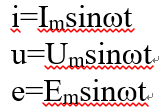2、最大值：

交变电流的最大值是指交变电流在一个周期内所能达到的最大值，它可以用来表示交变电流的强弱或电压的高低。以正弦交流电为例。则有：Em=nBωS，此时电路中的电流强度及用电器两端的电压都具有最大值，Im=Em/(R+r); Um=ImR

3、有效值：

交变电流的有效值是根据电流的热效应来定义的，让交变电流和恒定电流通过相同阻值的电阻，如果在相同的时间内产生的热量相等，我们就把这一恒定电流的数值叫做这一交变电流的有效值。

交流电的有效值是根据它的热效应确定的。交流电流i通过电阻R在一个周期内所产生的热量和直流电流I通过同一电阻R在相同时间内所产生的热量相等, 则这个直流电流I的数值叫做交流电流i的有效值, 用大写字母表示, 如I、 U等。

一个周期内直流电通过电阻R所产生的热量为：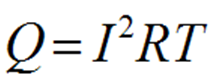交流电通过同样的电阻R，在一个周期内所产生热量：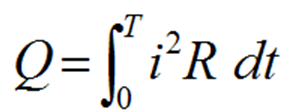根据定义，这两个电流所产生的热量应相等，即：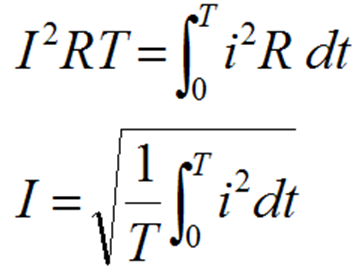将代入上式i=Imsinωt，得：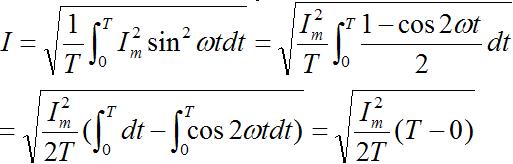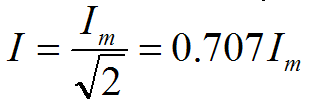4、平均值：

交变电流的平均值是指在某一段时间内产生的交变电流对时间的平均值。 对于某一段时间或某一过程，其平均感应电动势：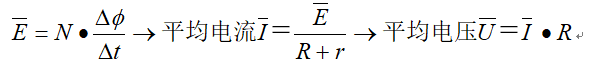二、正弦交流电的“四值”之间的关系 ：

1、正弦交流电的有效值与最大值的关系：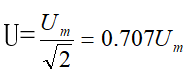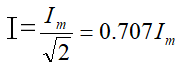注：I U 是电流、电压的有效值，Im、Um是电流、电压的最大值

2、正弦交流电的平均值与最大值和有效值的关系：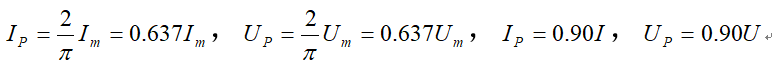注：Ip、Up是电流、电压的平均值

三、“四值”解题方法小结：

交变电流有四值，即有效值、平均值、最大值和瞬时值．各值何时应用，对照如下情况确定：

（1）在研究电容器是否被击穿时，要用峰值（最大值）．因电容器上标明的电压是它在直流电源下工作时所承受的最大值．

（2）在研究交变电流的功率和产生的热量时，只能用有效值．

（3）在求解某一时刻线圈受到的磁力矩时，只能用瞬时值．

（4）在求交变电流流过导体的过程中通过导体截面积的电量时，要用平均值．


展开全文• 理想电子管功放对输出变压器要求为: ( 1) 初级电感为无穷大, 以获得很低低频信号; ( 2) 漏感、分布电感、分布电容为零, 以便高保真地 ...且较大的初 级电感又可使相位失真加大, 动态范围减少。
• 仿真软件 - Comsol仿真模型 -...- 磁场https://www.zhihu.com/video/942396452278874112主要思想：将求解出来频域结果，手动添加时间项，即（求解出结果是包含幅值和初相位信息），然后添加时间参数，生成动画...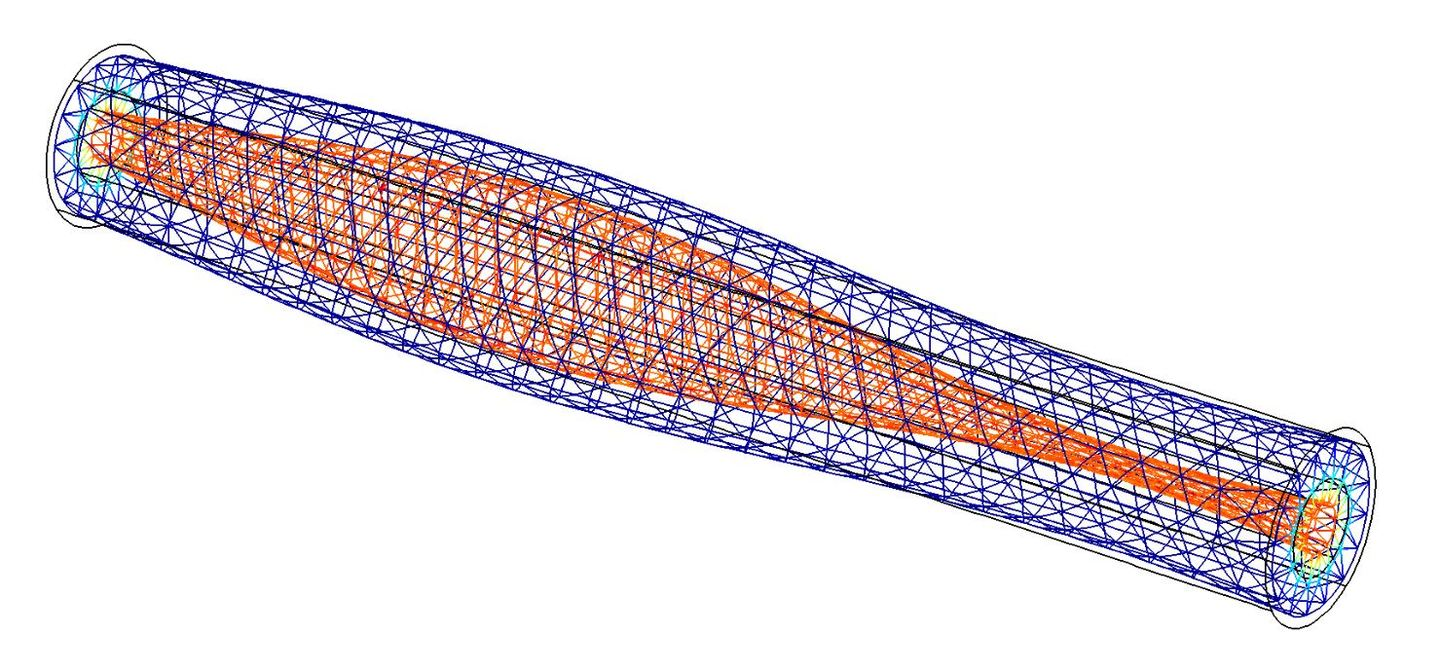仿真软件 - Comsol仿真模型 - 同轴电缆I. 同轴电缆 - 电场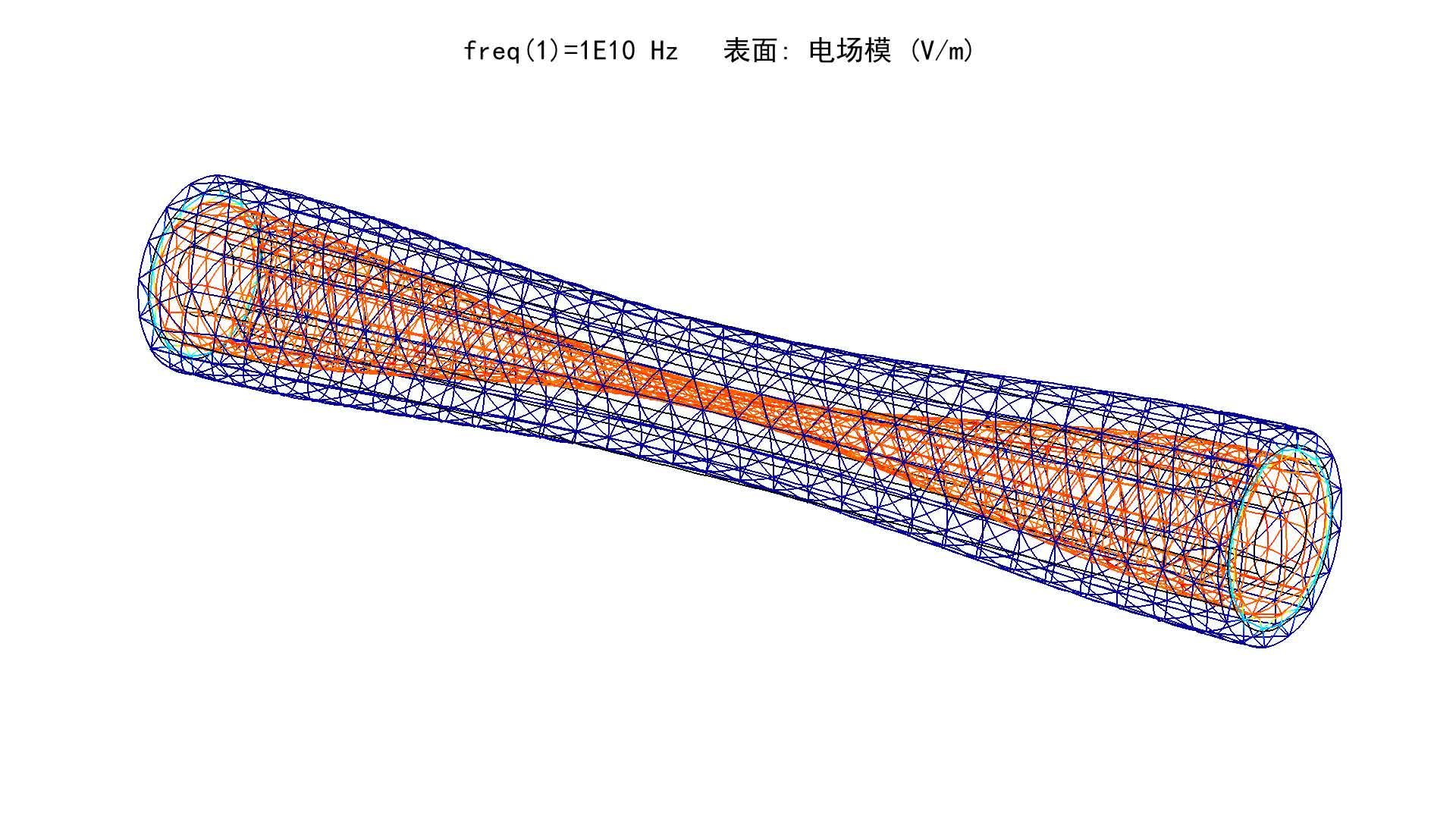https://www.zhihu.com/video/942396348398649344II. 同轴电缆 - 磁场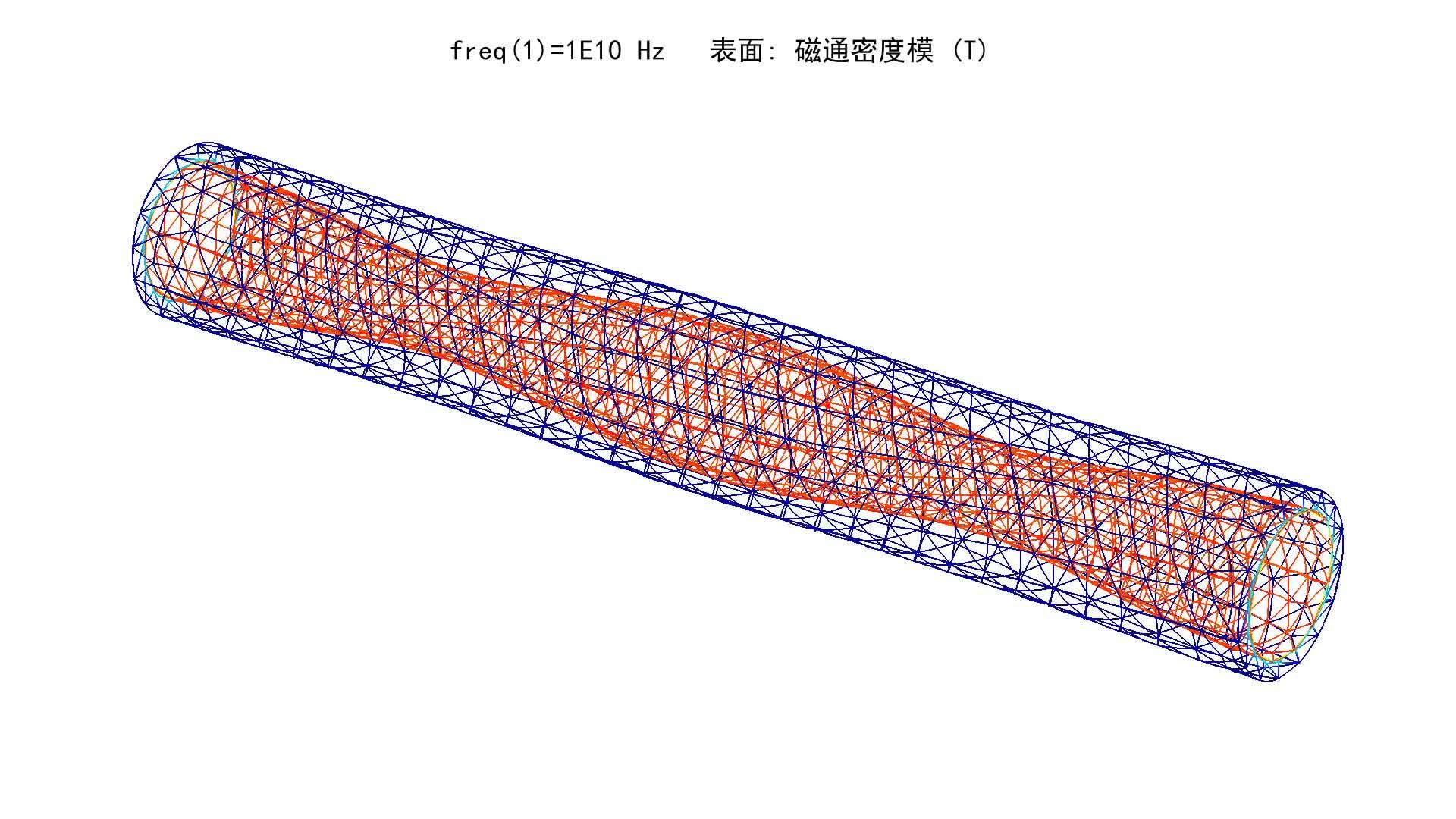https://www.zhihu.com/video/942396452278874112主要思想：将求解出来的频域结果，手动添加时间项，即（求解出的结果是包含幅值和初相位信息的），然后添加时间参数，生成动画的时候，对时间进行累加即可。COMSOL源文件以及动画gif文件 付费购买渠道：COMSOL 同轴电缆 - 磁场、电场动态图形​mianbaoduo.com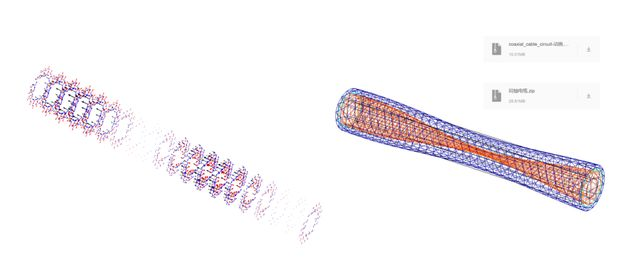关于作者目前电气工程博士在读，平常喜欢琢磨有限元仿真、Matlab、Latex、三维建模等一切有趣的东西一些成果会放在 Github 或者 知乎，也欢迎通过邮箱联系我 dongweihao514@sina.com
展开全文• 对液晶空间光调制器(LC-SLM)在读出光斜入射下理论进行了推导，建立了...随着入射角增加，读出光强的初相位逐渐减小，但该初相位值并不大，即使在入射角10°下达到最大，也仅为0.21π。计算结果与实验数据相吻合。
• 其次,根据加窗自相关信号初相位为零特点生成参考信号,实现参考信号与加窗自相关信号相位匹配;最后,根据柯西不等式,利用参考信号和加窗自相关信号构造反映参考信号和加窗自相关信号频率匹配程度误差函数,误差...
• 输入信号经过A/D变换得到数字信号,经过频率校正环节后可得到与输入信号基波分量相同角频率信号,该信号再经过相校正环节后就可以得到与输入信号基波分量同频率、同的相位信号,从而实现对输入信号中基波分量...有源滤波器
• 相量和向量区别 正弦量本身是没有方向标量，...相量与坐标轴夹角表示了正弦量的初相位，向量与坐标轴夹角则表示了其在空间中方向。 下面从数学运算角度，再对两者进行观察。 向量 坐标形式：由坐标系下各个
相量和向量的区别
正弦量本身是没有方向的标量，为了方便计算而引入相量这种工具，相量表现出了正弦量的有效值和相位；而表示力、电场强度、磁感应强度等的空间向量是有大小、有方向的矢量，箭头代表向量的方向，长度代表向量的大小。二者是有本质区别的。即相量本身是表示正弦量，向量本身则是表示既有大小又有方向的量。相量的模值是正弦量的有效值，向量的模值是表示量的大小。相量与坐标轴的夹角表示了正弦量的初相位，向量与坐标轴的夹角则表示了其在空间中的方向。
下面从数学运算的角度，再对两者进行观察。
向量
坐标形式：由坐标系下各个轴方向的基向量的大小$\vec{a}=x\vec{i}+y\vec{j}+z\vec{k}=\left ( x,y,z \right )$
加法：首尾相连，满足三角形法则。$\vec{a}+ \vec{b}=（x_{a}+x_{b},y_{a}+y_{b}）$
数量积（点乘）：$\vec{a}\cdot \vec{b}=\left |a \right |\left | b \right |\cos \left ( \vec{a},\vec{b} \right )$
向量积（叉乘）：$\vec{a}\times \vec{b}=\left |a \right |\left | b \right |\sin \left ( \vec{a},\vec{b} \right )\vec{e_{z}}$
相量
这里解释一点。正弦量用相量来表示的根由是，一个正弦量首先可以用一个复指数函数进行表示。我们平时常见到的复数是$a+bj=r\cos \theta +r\sin \theta j=re^{j\theta }$的形式。通过这个式子，可以发现复数和正弦量可以对应起来，即一个复数的实部就是复数的幅值乘以一个角度的余弦。当我们把复数中的$\theta$换成$\omega t+\varphi$，然后我们来看下会发生什么。
$re^{j(\omega t+\varphi)}=r\cos(\omega t+\varphi)+r\sin(\omega t+\varphi)j$$Re[re^{j(\omega t+\varphi)}]=r\cos(\omega t+\varphi)$
在只取实部的时候，就表示了一个角频率为$\omega$，幅值为$r$，初相位为$\varphi$的正弦量。在正弦量的运算中，频率都是保持一致，并取有效值。所以在这里可以略去$e^{j \omega t}$。即一个正弦量$i=\sqrt{2}I \cos(\omega t+\varphi)$可以用复数表示为$i=Re[\sqrt{2}Ie^{j\varphi}e^{j\omega t}]=Re[\sqrt{2}\dot{I}e^{j\omega t}]$。相量$\dot{I}=Ie^{j\varphi}$，其实相量也就是一个复数。所以相量的运算，其实也就是复数的运算，这一点和向量的运算是不同的。
坐标形式：$\dot{A}=Ae^{j\varphi_{a}}=a_{1}+ja_{2}$
加法：也满足三角形法则，$\dot{A}+\dot{B}=(a_{1}+b_{1})+j(a_{2}+b_{2})$
乘法：$\dot{A}\dot{B}=ABe^{j(\varphi_{a}+\varphi_{b})}$
除法：$\dot{A}/\dot{B}=ABe^{j(\varphi_{a}-\varphi_{b})}$
注意：相量运算这里没有点乘，叉乘的说法。复数的运算结果还是复数。而向量运算完之后（如点乘），则会变成标量。
正弦电路中的复功率
在正弦电路中，复功率的定义为：$\dot{S}=\dot{U}\dot{I^*}=UIe^{j(\varphi_{u}-\varphi_{i})}=UI\cos(\varphi_{u}-\varphi_{i})+jUI\sin(\varphi_{u}-\varphi_{i})$。相量说到底就是个复数，在这里复功率的实部表示有功功率，虚部表示无功功率。
电磁场中的坡印廷矢量
在电磁场中，反映空间中某点电磁能量流动的能力方向的矢量是坡印廷矢量，它是电磁能流的功率密度。表达式为：$\vec{S}=\vec{E}\times \vec{H}$。它的模值大小反映了单位时间内穿过与电磁能流方向垂直的单位面积的电磁能量，它的方向便是电磁能量流动的方向。当电场强度和磁通密度不随时间变化时，波印廷矢量也便是一个常矢量。
正弦电磁场中的复坡印廷矢量
之所以介绍这个。是因为复坡印廷矢量既是一个向量，又是一个相量，比较特殊。上面介绍的正弦电路中的复功率，只是一个相量，或者说只是一个复数比较好理解。而静态场中的坡印廷矢量为一个常矢量，不随时间变化，只是一个单纯的向量。下面针对正弦电磁场中的复坡印廷矢量进行分析。
首先可以看出来的是在正弦电磁场中的电场强度和磁通密度也都同时有了两重身份：既是向量，又是相量。首先空间中的电场强度E它既是空间位置的函数，还是时间的函数。如果说我选定空间中某一点，那么电场强度就只是时间的函数了。
$\vec{E}(t)=E_{xm}\cos(\omega t+\varphi_x)\vec{e_{x}}+E_{ym}\cos(\omega t+\varphi_y)\vec{e_{y}}+E_{zm}\cos(\omega t+\varphi_z)\vec{e_{z}}$$\vec{E}(t)=Re[\dot{E_{x}}\sqrt{2}e^{j\omega t} \vec{e_{x}}+\dot{E_{y}}\sqrt{2}e^{j\omega t} \vec{e_{y}}+\dot{E_{z}}\sqrt{2}e^{j\omega t} \vec{e_{z}}]=Re[\dot{\vec{E}}\sqrt{2}e^{j\omega t} ]$$\dot{\vec{E}}=\dot{E_{x}}\vec{e_{x}}+\dot{E_{y}} \vec{e_{y}}+\dot{E_{z}} \vec{e_{z}}$
所以在空间中该点处这时的电磁能流密度就等于
$\dot{\vec{S}}=\dot{\vec{E}} \times \dot{\vec{H}}$针对这个式子，首先考虑向量的叉乘，即$\dot{\vec{S}}=\dot{\vec{E}} \times \dot{\vec{H}}=|\dot{\vec{E}}||\dot{\vec{H}}|\sin \left ( \dot{\vec{E}},\dot{\vec{H}} \right )\vec{e_{z}}$。后面的正弦值是两个相量之间夹角的正弦，而前面部分则是两个向量的模值相乘。$\dot{\vec{E}}$和$\dot{\vec{H}}$本身在x，y，z三个方向上均有值。需要注意，这个地方写的只是向量叉乘的定义。即叉乘的定义，确定了两个向量叉乘之后向量的模值和方向。当知道向量的坐标后，可以直接在坐标系下进行叉乘的坐标运算，如下所示
$\dot{\vec{S}}=(\dot{E_{x}}\vec{e_{x}}+\dot{E_{y}} \vec{e_{y}}+\dot{E_{z}} \vec{e_{z}}) \times (\dot{H_{x}}\vec{e_{x}}+\dot{H_{y}} \vec{e_{y}}+\dot{H_{z}} \vec{e_{z}})=\begin{bmatrix} \vec{e_{x}}&\vec{e_{y}} &\vec{e_{z}} \\ \dot{E_{x}}& \dot{E_{y}} & \dot{E_{z}}\\ \dot{H_{x}}& \dot{H_{y}} & \dot{H_{z}} \end{bmatrix}$$\dot{\vec{S}}=\begin{bmatrix} \dot{E_{y}} & \dot{E_{z}}\\ \dot{H_{y}} & \dot{H_{z}} \end{bmatrix}\vec{e_x}+\begin{bmatrix} \dot{E_{z}} & \dot{E_{x}}\\ \dot{H_{z}} & \dot{H_{x}} \end{bmatrix}\vec{e_y}+\begin{bmatrix} \dot{E_{x}} & \dot{E_{y}}\\ \dot{H_{x}} & \dot{H_{y}} \end{bmatrix}\vec{e_z}$$\dot{\vec{S}}=(\dot{E_{y}}\dot{H_{z}}-\dot{E_{z}}\dot{H_{y}})\vec{e_x}+(\dot{E_{z}}\dot{H_{x}}-\dot{E_{x}}\dot{H_{z}})\vec{e_y}+(\dot{E_{x}}\dot{H_{y}}-\dot{E_{y}}\dot{H_{x}})\vec{e_z}$
到这一步，可以看到在进行了向量的叉乘坐标运算后，叉乘所得向量的每一个分量都是两个相量相乘然后做差。也就是说最终复坡印廷矢量的三个分量，还是由电场强度、磁通密度各部分分量相量相乘之后得到的。实际上电场强度$\dot{\vec{E}}$（大小和相位）由电压$\dot{U}$（标量，或者说电势）所决定，磁通密度$\dot{\vec{B}}$（大小和相位）由电流$\dot{I}$所决定。而$\dot{U}$和$\dot{I}$两个复数之间的相角差，决定了有功、无功的大小分配。所以$\dot{\vec{E}}$和$\dot{\vec{H}}$各个分量复数之间的相角差，也同样代表了功率因数角。故实际的复坡印廷矢量的表达式为
$\dot{\vec{S}}=\dot{\vec{E}} \times \dot{\vec{H^*}}$它的实部就是坡印廷矢量的平均值（或有功功率密度），表示能量的流动；虚部是无功功率密度，表示着电磁能量的交换。


展开全文• 利用滑窗离散傅里叶变换方法检测系统剩余谐波电流的幅值和初相位，并给出了控制策略的参数确定方法和校正信号的计算方法。分别仿真分析了不同控制参数下、有无背景谐波情况下和负载不同变化剧烈程度下的滤波效果，并...
• 通过对冷原子干涉仪近似数学模型变换，研究了原子速度与跃迁概率之间关系，阐述了基于辅助角速度测量原子速度扫描法测量原理。在原子干涉仪精确模型基础上，利用Raman 激光频率调制对多普勒效应进行补偿，...
• 静态系统Matlab编程仿真 静态系统：也称为无记忆系统。一般地，无记忆系统数学描述是代数方程（组）。 静态系统仿真过程就是相应代数方程数值...所有余弦波的初相位为0。 建立数学模型： 根据题设，该调...
静态系统的Matlab编程仿真
静态系统：也称为无记忆系统。一般地，无记忆系统的数学描述是代数方程（组）。
静态系统的仿真过程就是相应的代数方程的数值计算或求解过程。
试仿真得出一个幅度调制系统的输入输出波形。设输入被调制信号是一个幅度为2v，频率为1000Hz的余弦波，调制度为0.5，调制载波信号是一个幅度为5v，频率为10KHz的余弦波。所有余弦波的初相位为0。
建立数学模型：
根据题设，该调幅系统的输入输出关系表达式为：
$y(t)=(M+m_aMcos2πf_mt)×Acos2πf_ct\,$
其中，$M=2$是被调信号的振幅，$f_m$=1000是其频率。$A=5$是载波信号的幅度，$f_c=10^4$是其频率。$m_a=0.5$是调制度。
编程实现:
连续函数必须进行离散化才能够存储于计算机中。只要时间离散化过程满足取样定理，那么就不会引起失真。在这个系统中的信号最高工作频率为$(f_m+f_c)=11KHz$，根据取样定理，只要离散取样率高于该频率的2倍即可无失真。在计算量和数据存储量许可的条件下，取样率可以设置更高，以使仿真计算的结果波形图显示更加光滑。这里，我们设置取样率为$10^5$，即在一个载波周期上取样10次，相应的取样间隔为$Δt=10^{-5}s$。本例中，取样间隔也作为仿真步进。程序代码如下:
dt = 1e-5;                              % 仿真采样间隔
T = 3*1e-3;                             % 仿真终止时间
t = 0:dt:T                              % 时间从0开始到T结束，采样间隔为dt
input = 2 * cos(2 * pi * 1000 * t);     % 输入信号
carrier = 5*cos(2 * pi * 10000 * t);    % 载波信号
output = (2 + 0.5 * input).*carrier;    % 输出信号
% 作图。将图分成 3 个区域。
subplot(3,1,1); plot(t,input);xlabel('时间 t');ylabel('输入信号');
subplot(3,1,2); plot(t,carrier);xlabel('时间 t');ylabel('载波信号');
subplot(3,1,3); plot(t,output);xlabel('时间 t');ylabel('输出信号');

运行结果：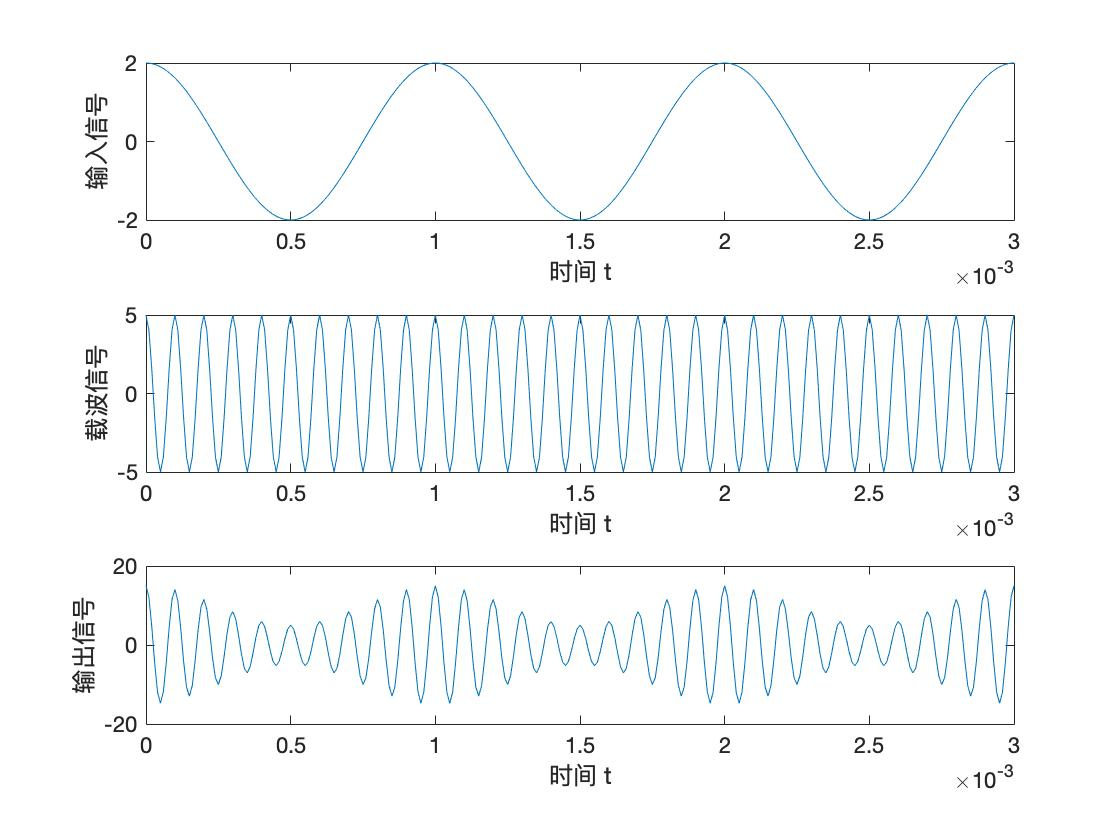展开全文• 电流有效值Ts -采样间隔 -电流相角 下面几种算法都是假定被采样电压电流信号都是纯正弦函数既不含非周期分量又不含谐波分量因而可利用正弦函数种种特性从若干个离散化采样值中计算出电流电压幅值相位角和...
• 简要介绍了奇异值分解（SVD）滤波与幅度相位估计（APES）算法原理，...转子断条故障试验结果表明：对于短时信号，所提方法仍能较精确地识别定子电流中故障分量频率，并准确计算其幅值和相角，且不受噪声影响。
• 2)载波：给定幅度正弦波，为简单起见，初相位设为0，频率为550k~1605kHz 内可调。接收机选频放大滤波器带宽为12KHz ，中心频率为1000kHz 。 在信道中加入噪声。当调制度为0.3时，设计接收机选频滤波器输出信噪比为...
• 在振动频率不超过500 Hz情况下,仿真计算了振幅、频率、初相位和峰值加速度等振动参数对SAL图像分辨率影响,给出了振动影响下理想点目标和扩展面目标SAL图像。仿真结果表明,振动峰值加速度是直接和图像模糊...
• 文中提出基于Kaiser窗的三谱线插值FFT的电力谐波分析方法，推导了基波以及各次谐波的幅值、频率和初相位的插值修正公式。仿真结果表明，所提算法设计灵活且易于实现，在基波频率波动以及白噪声干扰下都有较高的谐波...
• 一个正弦量具有幅值、频率及初相位三要素。 这些特征可用一些方法表示出来。 正弦量各种表示方法是分析与计算正弦交流电路工具。   前面已讲过两种表示法。 用三角函数式来表示,i=Imsin⁡wti= I_m \sin wti=...
• 载波：给定幅度正弦波，为简单起见初相位设为0，频率为550KHz到1605KHz可调。 接收机选频滤波器带宽为12KHz，中心频率为1000KHz。 在信道中加入噪声。当调制度为0.3时，设计接收机选频滤波器输出信噪比为20dB，...
• 具有弱场分布情况下的波导的辐射场的计算均提供了一个简单的方法。 论文第五章改进了镜面系统的数值优化过程，将相位校正镜面的加工精度 对转换效率的影响引入了数值优化中。使用优化后的准光学模式转换器在回旋 管...
• 题意：给出边界条件和球半径，给出初始球心位置和...思路：第一次做计算几何题，有种高中还是初中题感觉，比如这题好像以前三月考做过但是没有想起来。用翻折思想，看代码瞬间恍然大悟。网上题解有用eps =
• 第七章里叙述了倒谱和同态算法，侧重于同态系统相位计算的难点。 第八章里给出了有关内插和抽取几个程序。 本书是在1976年，由IEEE声学、语言学以及信号处理学会所组成数字信号处理委员会，着手进行一项...
• 第七章里叙述了倒谱和同态算法，侧重于同态系统相位计算的难点。 第八章里给出了有关内插和抽取几个程序。 本书是在1976年，由IEEE声学、语言学以及信号处理学会所组成数字信号处理委员会，着手进行一项...
• 第七章里叙述了倒谱和同态算法，侧重于同态系统相位计算的难点。 第八章里给出了有关内插和抽取几个程序。 本书是在1976年，由IEEE声学、语言学以及信号处理学会所组成数字信号处理委员会，着手进行一项...
• 第七章里叙述了倒谱和同态算法，侧重于同态系统相位计算的难点。 第八章里给出了有关内插和抽取几个程序。 本书是在1976年，由IEEE声学、语言学以及信号处理学会所组成数字信号处理委员会，着手进行一项...
• 4.2.1 哈密顿系统的初积分 4.2.2 Lax Pair和哈密顿系统 4.2.3 Floquet理论 4.3 哈密顿混沌 4.3.1 轨迹和Henon?Heiles哈密顿函数 4.3.2 表面分割法 第5章 非线性耗散系统 5.1 不动点和稳定性 5.2 轨迹 5.3 相位图 5.4...